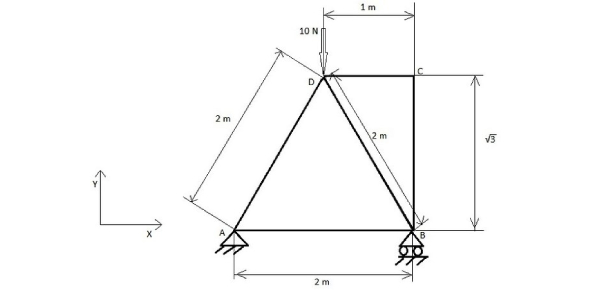# Ansys Structural Analysis Quiz

20 Questions | Attempts: 3740
ShareSettingsAre you a student of software? Here we bring an Ansys structural analysis quiz for you. Ansys is an American-based company that develops and markets CAE/multiphysics engineering simulation software for the purpose of product design, testing, and operation. Here, we will see how well you understand Ansys structural analysis and some other things. Try to answer as many questions as you can to get a good score. Let's go for it then.

• 1.
No. of degrees of freedom for 3D Solid Element (both Hex and Tet) ......
• A.

3

• B.

2

• C.

6

• D.

8

• 2.
Large deflection option when switched ON accounts for which of the following:
• A.

Stiffness variation

• B.

Large rotations

• C.

Large strains

• D.

All of the above

• 3.
ANSYS archive files are saved in...............format.
• A.

.wbpj

• B.

.scdoc

• C.

.wbpz

• D.

.cmdp

• 4.
Which numerical method is used by ANSYS to converge a nonlinear solution?
• A.

Euler Method

• B.

Bisection Method

• C.

Taylor's Method

• D.

Newton-Raphson Method

• 5.
Stresses and strains calculated on an element at.............
• A.

Nodes

• B.

Integration points

• C.

Element center

• D.

All of the above

• 6.
Which of these loads are NOT supported in Harmonic Analysis?
• A.

• B.

Pressure

• C.

Moment

• D.

All of the above

• 7.
How many degrees of freedom exist for a cylindrical joint?
• A.

2

• B.

3

• C.

4

• D.

6

• 8.
What are the mandatory material properties needed for Static Structural Analysis?
• A.

Young's Modulus and Yield strength

• B.

Young's Modulus and Poisson's ratio

• C.

Yield Strength and Poisson's ratio

• D.

Ultimate strength and Yield Strength

• 9.
In ANSYS meshing, what does the pinch option in the local mesh control do?
• A.

Generate prism layer near free surfaces

• B.

Remove intricate & small features.

• C.

Create a structured mesh on a face

• D.

Move nodes around a local region

• 10.
Possible to copy (Ctrl +C) and paste (ctrl + V) any geometrical entities such as curves, faces, and bodies in SpaceClaim.
• A.

True

• B.

False

• 11.
Both the Pull Tool and Move Tool can be used to extrude a face.
• A.

True

• B.

False

• 12.
A body suppressed in SpaceClaim will be imported into Mechanical.
• A.

True

• B.

False

• 13.
Acceleration can be applied to a single individual part of an assembly.
• A.

True

• B.

False

• 14.
Results from Random Vibration Analysis are frequency dependent.
• A.

True

• B.

False

• 15.
FEM gives an exact solution to problems.
• A.

True

• B.

False

• 16.
What does an eigenvector represent in the formulation of Modal Analysis?
• A.

Natural frequency

• B.

Forcing frequency

• C.

Mode shape

• D.

Phase angle

• 17.
Which of these is NOT a solver in ANSYS?
• A.

PCG Solver

• B.

Direct Solver

• C.

Symmetric Solver

• D.

Iterative Solver

• 18.
Which of the following can be used to change the status of a contact pair to CLOSE in the Mechanical window?
• A.

Interference removal

• B.

Pinball region

• C.

Through the contact detection Method

• D.

All of the above

• 19.
APDL solver and Workbench solver are two separate independent solvers.
• A.

True

• B.

False

• 20.
When frictionless contact type is assigned to a particular contact interface in modal analysis, ANSYS will automatically convert it into a no separation type contact.
• A.

True

• B.

False

## Related TopicsBack to top
×

Wait!
Here's an interesting quiz for you.